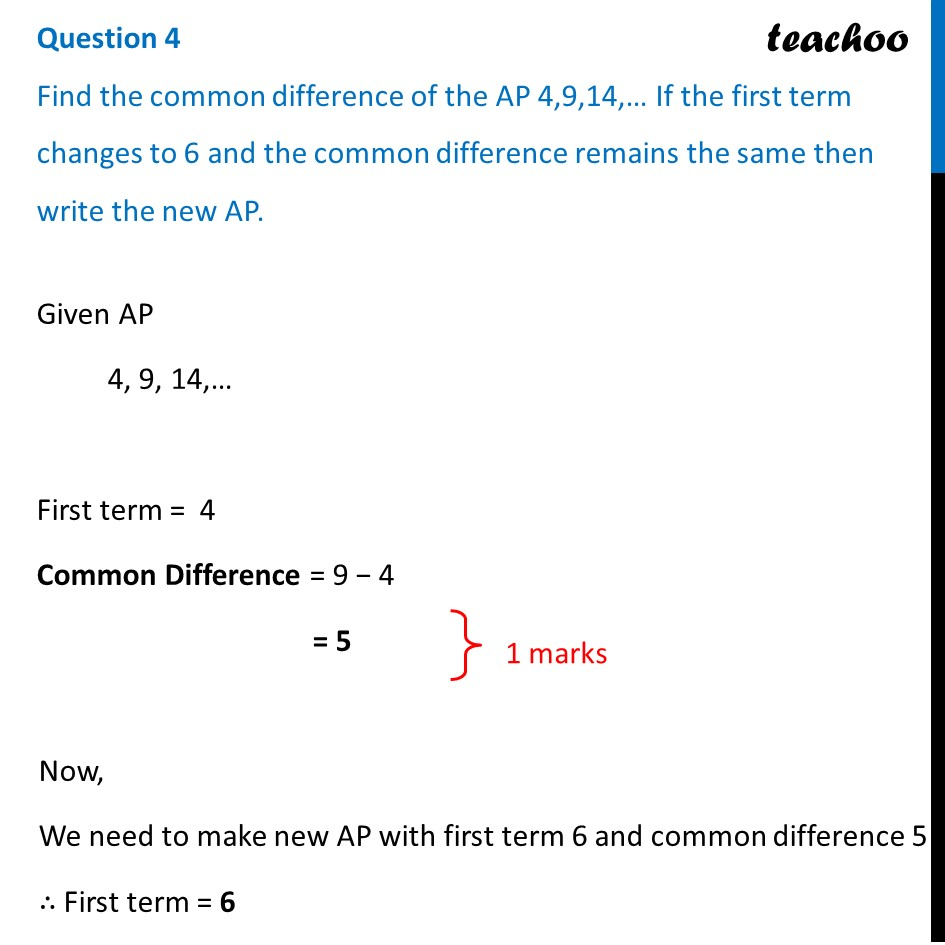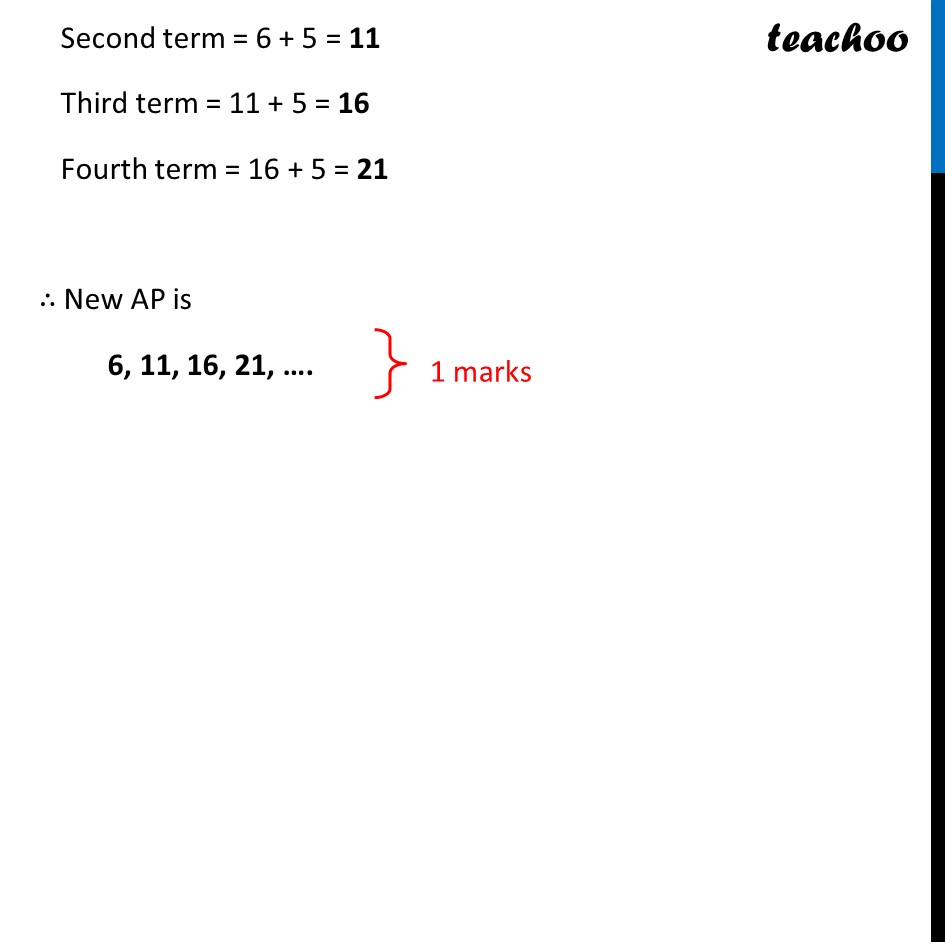CBSE Class 10 Sample Paper for 2022 Boards - Maths Basic [Term 2]

Class 10
Solutions of Sample Papers for Class 10 Boards

## Find the common difference of the AP 4,9,14,… If the first term changes to 6 and the common difference remains the same then write the new AP.Get live Maths 1-on-1 Classs - Class 6 to 12

### Transcript

Find the common difference of the AP 4,9,14,… If the first term changes to 6 and the common difference remains the same then write the new AP. Given AP 4, 9, 14,… First term = 4 Common Difference = 9 − 4 = 5 Now, We need to make new AP with first term 6 and common difference 5 ∴ First term = 6 Second term = 6 + 5 = 11 Third term = 11 + 5 = 16 Fourth term = 16 + 5 = 21 ∴ New AP is 6, 11, 16, 21, ….